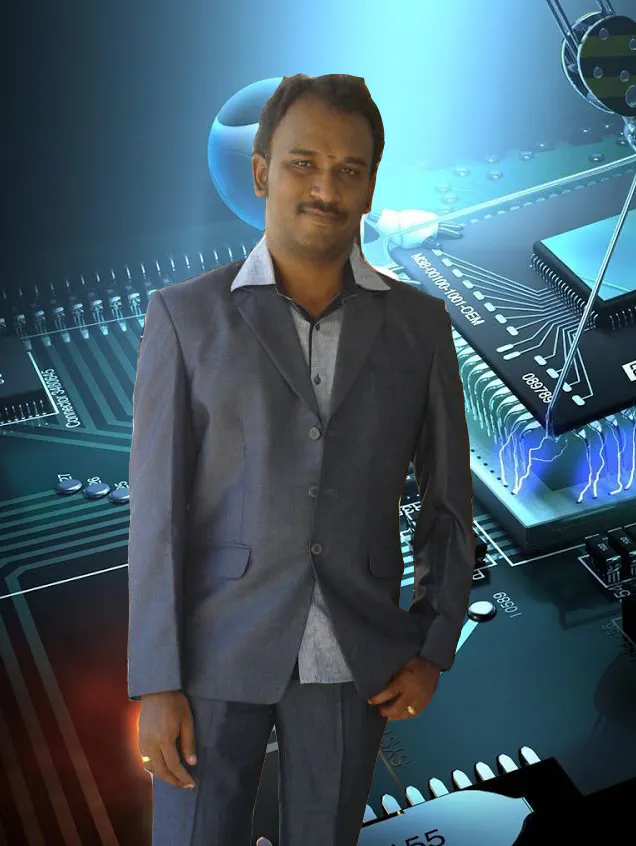# Trash Built BT Line Drawing Bot - My Bot

Till the completion of Cute Drawing Buddy V1, SCARA Robot - Arduino I plan for a another drawing bot, main aim is to cover a large space.

IntermediateFull instructions provided2 days1,586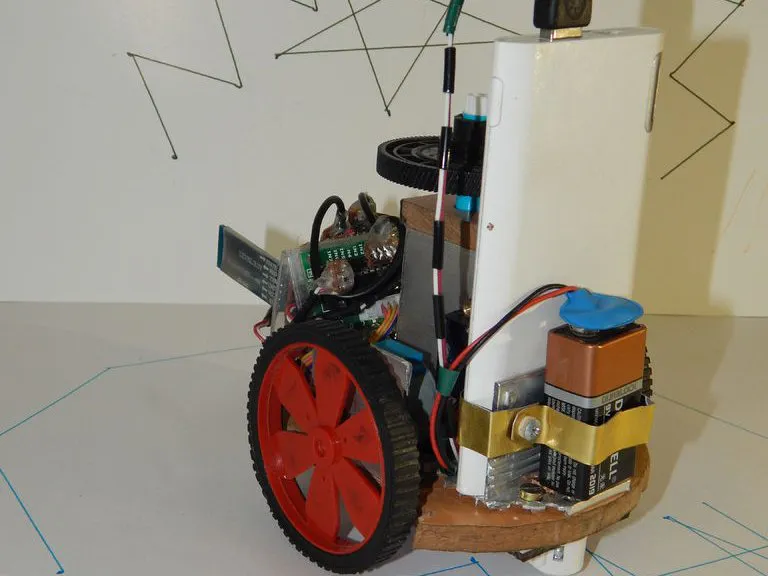## Things used in this project

### Hardware componentsArduino Nano R3
×1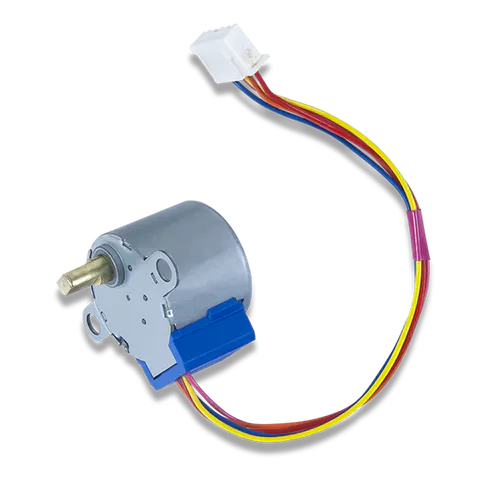Digilent Stepper Motor
×1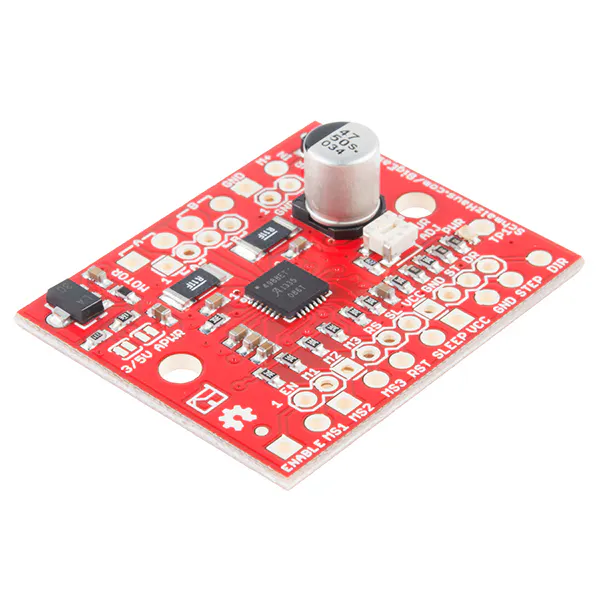SparkFun Stepper motor driver board A4988
×1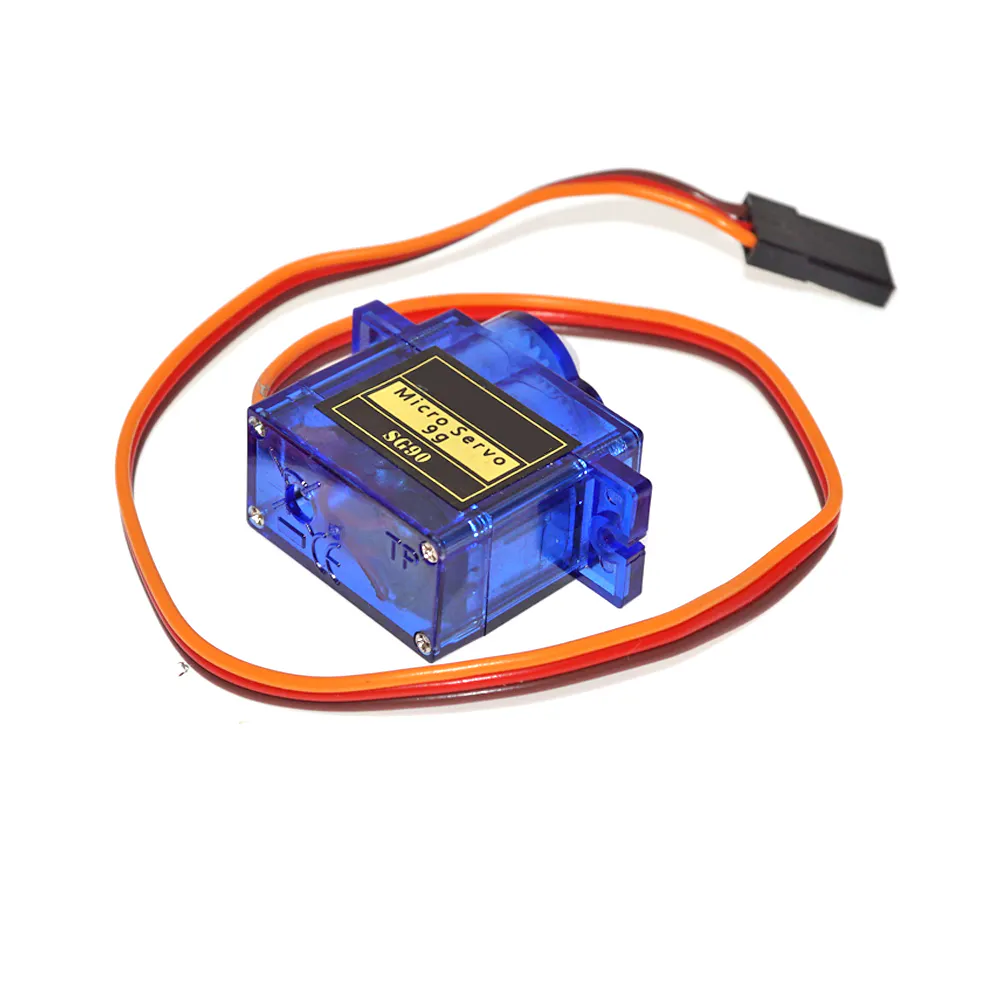SG90 Micro-servo motor
×1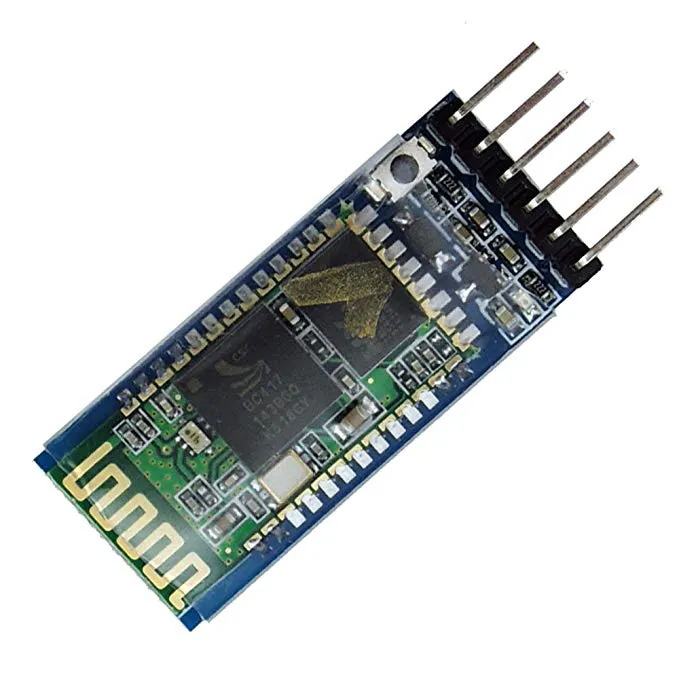HC-05 Bluetooth Module
×1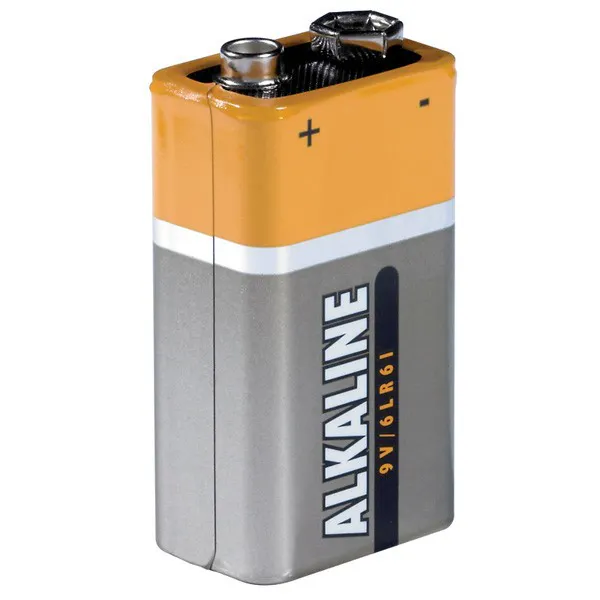9V battery (generic)
×1
 powerbank
×1

### Software apps and online servicesArduino IDE
 VisualStudio 2010

### Hand tools and fabrication machinesSoldering iron (generic)
 Hack Saw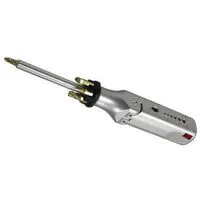Multitool, Screwdriver

## Schematics

### Circuit Diagram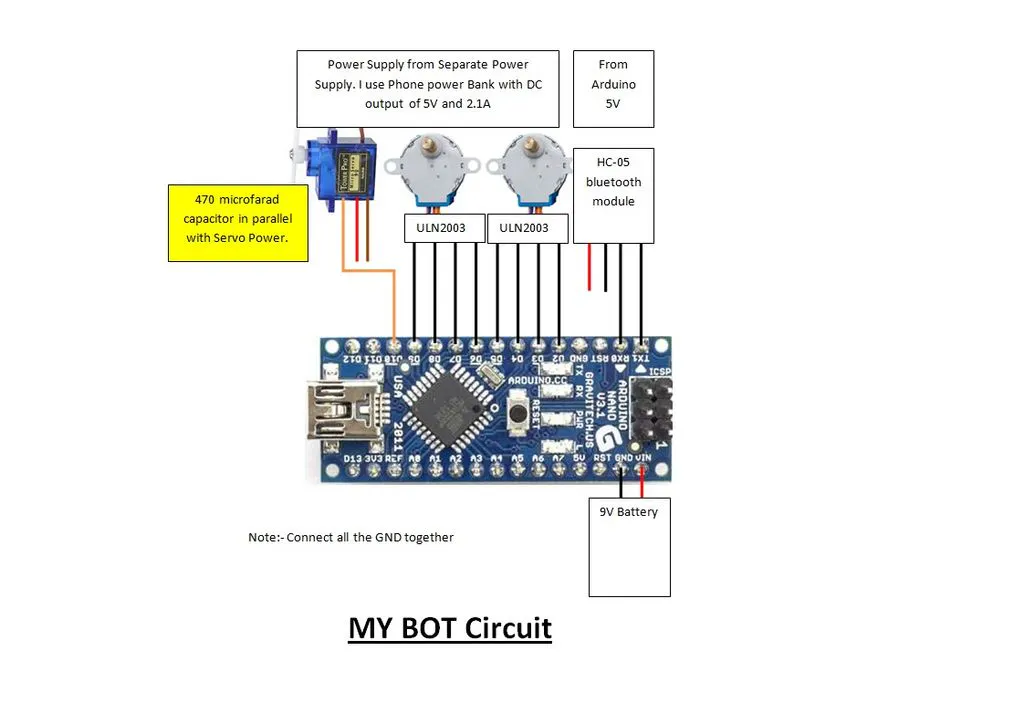## Code

### Check.ino

Arduino
```char command = 'S';

boolean Direction1 = false;
boolean Direction2 = true;

int steps = 0;
int Steps1 = 0;
int Steps2 = 0;

//Stepper1
#define IN11  6
#define IN12  7
#define IN13  8
#define IN14  9

//Stepper2
#define IN21  2
#define IN22  3
#define IN23  4
#define IN24  5

void setup()
{
Serial.begin(9600);

pinMode(IN11, OUTPUT);
pinMode(IN12, OUTPUT);
pinMode(IN13, OUTPUT);
pinMode(IN14, OUTPUT);
pinMode(IN21, OUTPUT);
pinMode(IN22, OUTPUT);
pinMode(IN23, OUTPUT);
pinMode(IN24, OUTPUT);
}

void loop(){
if(Serial.available() > 0){
}

if(command=="F")
{
Direction1=1;
Direction2=0;
}
else if(command=="B")
{
Direction1=0;
Direction2=1;
}
else if(command=="L")
{
Direction1=0;
Direction2=0;
}
else if(command=="R")
{
Direction1=1;
Direction2=1;
}
if (command != "S")
{
SetDirection1();
SetDirection2();
}
delay(10);
}

void stepper1(){
switch(Steps1){
case 0:
digitalWrite(IN11, LOW);
digitalWrite(IN12, LOW);
digitalWrite(IN13, LOW);
digitalWrite(IN14, HIGH);
break;
case 1:
digitalWrite(IN11, LOW);
digitalWrite(IN12, LOW);
digitalWrite(IN13, HIGH);
digitalWrite(IN14, HIGH);
break;
case 2:
digitalWrite(IN11, LOW);
digitalWrite(IN12, LOW);
digitalWrite(IN13, HIGH);
digitalWrite(IN14, LOW);
break;
case 3:
digitalWrite(IN11, LOW);
digitalWrite(IN12, HIGH);
digitalWrite(IN13, HIGH);
digitalWrite(IN14, LOW);
break;
case 4:
digitalWrite(IN11, LOW);
digitalWrite(IN12, HIGH);
digitalWrite(IN13, LOW);
digitalWrite(IN14, LOW);
break;
case 5:
digitalWrite(IN11, HIGH);
digitalWrite(IN12, HIGH);
digitalWrite(IN13, LOW);
digitalWrite(IN14, LOW);
break;
case 6:
digitalWrite(IN11, HIGH);
digitalWrite(IN12, LOW);
digitalWrite(IN13, LOW);
digitalWrite(IN14, LOW);
break;
case 7:
digitalWrite(IN11, HIGH);
digitalWrite(IN12, LOW);
digitalWrite(IN13, LOW);
digitalWrite(IN14, HIGH);
break;
default:
digitalWrite(IN11, LOW);
digitalWrite(IN12, LOW);
digitalWrite(IN13, LOW);
digitalWrite(IN14, LOW);
break;
}
}

void stepper2(){
switch(Steps2){
case 0:
digitalWrite(IN21, LOW);
digitalWrite(IN22, LOW);
digitalWrite(IN23, LOW);
digitalWrite(IN24, HIGH);
break;
case 1:
digitalWrite(IN21, LOW);
digitalWrite(IN22, LOW);
digitalWrite(IN23, HIGH);
digitalWrite(IN24, HIGH);
break;
case 2:
digitalWrite(IN21, LOW);
digitalWrite(IN22, LOW);
digitalWrite(IN23, HIGH);
digitalWrite(IN24, LOW);
break;
case 3:
digitalWrite(IN21, LOW);
digitalWrite(IN22, HIGH);
digitalWrite(IN23, HIGH);
digitalWrite(IN24, LOW);
break;
case 4:
digitalWrite(IN21, LOW);
digitalWrite(IN22, HIGH);
digitalWrite(IN23, LOW);
digitalWrite(IN24, LOW);
break;
case 5:
digitalWrite(IN21, HIGH);
digitalWrite(IN22, HIGH);
digitalWrite(IN23, LOW);
digitalWrite(IN24, LOW);
break;
case 6:
digitalWrite(IN21, HIGH);
digitalWrite(IN22, LOW);
digitalWrite(IN23, LOW);
digitalWrite(IN24, LOW);
break;
case 7:
digitalWrite(IN21, HIGH);
digitalWrite(IN22, LOW);
digitalWrite(IN23, LOW);
digitalWrite(IN24, HIGH);
break;
default:
digitalWrite(IN21, LOW);
digitalWrite(IN22, LOW);
digitalWrite(IN23, LOW);
digitalWrite(IN24, LOW);
break;
}
}

void SetDirection1(){
if(Direction1==1){ Steps1++;}
if(Direction1==0){ Steps1--; }
if(Steps1>7){Steps1=0;}
if(Steps1<0){Steps1=7; }
stepper1();
}
void SetDirection2(){
if(Direction2==1){ Steps2++;}
if(Direction2==0){ Steps2--; }
if(Steps2>7){Steps2=0;}
if(Steps2<0){Steps2=7; }
stepper2();
}
```

### Mybotvector.ino

Arduino
```#include <Servo.h>
#include <EEPROM.h>
//Stepper1
#define IN11  6
#define IN12  7
#define IN13  8
#define IN14  9

//Stepper2
#define IN21  2
#define IN22  3
#define IN23  4
#define IN24  5

Servo servopen;
int steps = 0;
int Steps1 = 0;
int Steps2 = 0;
int movealternate=0;
double perdegree=15.95; //5750
double perstep=12; //4096
int rotatesteps;
int movesteps;
int recsteps;

int uppos = 25;
int downpos = 1;
String penstatus="U";
int movepen;
int servodelay=500;

boolean Direction1 = false;
boolean Direction2 = true;
//dir1=1 dir2=0 goes backwards
//dir1=1 dir2=1 turns right
//dir1=0 dir2=0 turns left
//dir1=0 dir2=1 goes forward

unsigned long last_time;
unsigned long currentMillis ;
long time;

String recstr = "";
String laststr = "";
String movec = "F";
String inputString;

char Incomvalue;
boolean stringComplete = false;

String S1;
String S2;
String Splitstr;
int Sstr1;
int Sstr2;
int findcomma;
int previouscomma;
boolean comp = true;

void setup()
{
Serial.begin(9600);
pinMode(IN11, OUTPUT);
pinMode(IN12, OUTPUT);
pinMode(IN13, OUTPUT);
pinMode(IN14, OUTPUT);
pinMode(IN21, OUTPUT);
pinMode(IN22, OUTPUT);
pinMode(IN23, OUTPUT);
pinMode(IN24, OUTPUT);

servopen.attach(10);
penstatus="D";
//  Serial.println("START");
if (penstatus=="D")
{
delay(servodelay);
servopen.write(uppos);
delay(servodelay);
//    for(movepen=downpos;movepen<=uppos;movepen++)
//    {
//      servopen.write(movepen);
//      delay(10);
//    }
penstatus="U";
}

laststr="S";
}
void loop()
{
if(stringComplete == true && comp == false)
{
do {
findcomma=0;
findcomma=S2.indexOf(",",findcomma);
if (findcomma<0)
{
recstr=S2;
executecmd();
sendcomp();
comp=true;
}
else
{
int strl = S2.length();
Splitstr=S2.substring(0,findcomma);
previouscomma=findcomma+1;
S2 = S2.substring(previouscomma);
recstr=Splitstr;
executecmd();
delay(10);
}
}
while (comp==false);
recstr="";
}
delay(1);
}

void executecmd()
{
if(recstr.substring(0,1)=="U")
{
if (penstatus=="D")
{
delay(servodelay);
servopen.write(uppos);
delay(servodelay);
penstatus="U";
}
delay(100);
}
else if(recstr.substring(0,1)=="D")
{
if (penstatus=="U")
{
delay(servodelay);
servopen.write(downpos);
delay(servodelay);
penstatus="D";
}
delay(100);
}
else if(recstr.substring(0,1)=="F")
{
Direction1=1;
Direction2=0;
getsteps();
steps=1;
movesteps=perstep*recsteps;
while(steps<movesteps){
SetDirection1();
SetDirection2();
steps++;
delay(1);
}
}
else if(recstr.substring(0,1)=="B")
{
Direction1=0;
Direction2=1;
getsteps();
steps=1;
movesteps=perstep*recsteps;
while(steps<movesteps){
SetDirection1();
SetDirection2();
steps++;
delay(1);
}
}
else if(recstr.substring(0,1)=="L")
{
Direction1=0;
Direction2=0;
steps=1;
getsteps();
rotatesteps=perdegree*recsteps;
while(steps<rotatesteps){
SetDirection1();
SetDirection2();
steps++;
delay(1);
}
}
else if(recstr.substring(0,1)=="R")
{
Direction1=1;
Direction2=1;
steps=1;
getsteps();
rotatesteps=perdegree*recsteps;
while(steps<rotatesteps){
SetDirection1();
SetDirection2();
steps++;
delay(1);
}
}
else if(recstr!="")
{
Serial.print("ERROR");
delay(50);
}
}

void getsteps()
{
recsteps=recstr.substring(1).toInt();
delay(50);
}

void sendcomp()
{
Serial.print("@");
delay(50);
stringComplete = false;
}

void stepper1(){
switch(Steps1){
case 0:
digitalWrite(IN11, LOW);
digitalWrite(IN12, LOW);
digitalWrite(IN13, LOW);
digitalWrite(IN14, HIGH);
break;
case 1:
digitalWrite(IN11, LOW);
digitalWrite(IN12, LOW);
digitalWrite(IN13, HIGH);
digitalWrite(IN14, HIGH);
break;
case 2:
digitalWrite(IN11, LOW);
digitalWrite(IN12, LOW);
digitalWrite(IN13, HIGH);
digitalWrite(IN14, LOW);
break;
case 3:
digitalWrite(IN11, LOW);
digitalWrite(IN12, HIGH);
digitalWrite(IN13, HIGH);
digitalWrite(IN14, LOW);
break;
case 4:
digitalWrite(IN11, LOW);
digitalWrite(IN12, HIGH);
digitalWrite(IN13, LOW);
digitalWrite(IN14, LOW);
break;
case 5:
digitalWrite(IN11, HIGH);
digitalWrite(IN12, HIGH);
digitalWrite(IN13, LOW);
digitalWrite(IN14, LOW);
break;
case 6:
digitalWrite(IN11, HIGH);
digitalWrite(IN12, LOW);
digitalWrite(IN13, LOW);
digitalWrite(IN14, LOW);
break;
case 7:
digitalWrite(IN11, HIGH);
digitalWrite(IN12, LOW);
digitalWrite(IN13, LOW);
digitalWrite(IN14, HIGH);
break;
default:
digitalWrite(IN11, LOW);
digitalWrite(IN12, LOW);
digitalWrite(IN13, LOW);
digitalWrite(IN14, LOW);
break;
}
}

void stepper2(){
switch(Steps2){
case 0:
digitalWrite(IN21, LOW);
digitalWrite(IN22, LOW);
digitalWrite(IN23, LOW);
digitalWrite(IN24, HIGH);
break;
case 1:
digitalWrite(IN21, LOW);
digitalWrite(IN22, LOW);
digitalWrite(IN23, HIGH);
digitalWrite(IN24, HIGH);
break;
case 2:
digitalWrite(IN21, LOW);
digitalWrite(IN22, LOW);
digitalWrite(IN23, HIGH);
digitalWrite(IN24, LOW);
break;
case 3:
digitalWrite(IN21, LOW);
digitalWrite(IN22, HIGH);
digitalWrite(IN23, HIGH);
digitalWrite(IN24, LOW);
break;
case 4:
digitalWrite(IN21, LOW);
digitalWrite(IN22, HIGH);
digitalWrite(IN23, LOW);
digitalWrite(IN24, LOW);
break;
case 5:
digitalWrite(IN21, HIGH);
digitalWrite(IN22, HIGH);
digitalWrite(IN23, LOW);
digitalWrite(IN24, LOW);
break;
case 6:
digitalWrite(IN21, HIGH);
digitalWrite(IN22, LOW);
digitalWrite(IN23, LOW);
digitalWrite(IN24, LOW);
break;
case 7:
digitalWrite(IN21, HIGH);
digitalWrite(IN22, LOW);
digitalWrite(IN23, LOW);
digitalWrite(IN24, HIGH);
break;
default:
digitalWrite(IN21, LOW);
digitalWrite(IN22, LOW);
digitalWrite(IN23, LOW);
digitalWrite(IN24, LOW);
break;
}
}

void SetDirection1(){
if(Direction1==1){ Steps1++;}
if(Direction1==0){ Steps1--; }
if(Steps1>7){Steps1=0;}
if(Steps1<0){Steps1=7; }
stepper1();
}
void SetDirection2(){
if(Direction2==1){ Steps2++;}
if(Direction2==0){ Steps2--; }
if(Steps2>7){Steps2=0;}
if(Steps2<0){Steps2=7; }
stepper2();
}

void serialEvent() {
while (Serial.available()) {
if (inChar == '\$') {
if (inputString == "Hai"){
Serial.print("3");
inputString="";
stringComplete = true;
}
else
{
S1=inputString + "\$";
inputString="";
Sstr1=0;
Sstr2=0;
Sstr1=S1.lastIndexOf("&");
Sstr2=S1.lastIndexOf("\$");
if(Sstr1>=0 && Sstr2>=0)
{
S2 = S1.substring(Sstr1+1,Sstr2);
comp = false;
previouscomma=0;
stringComplete = true;
recstr.trim();
recstr=S2;
Serial.print("\$");
}
else
{
Serial.print("#");
}
}
}
else
{
inputString += inChar;
}
}
}
```

## Credits

### jegatheesan

18 projects • 53 followers
Simply A Mechatronics Lover.